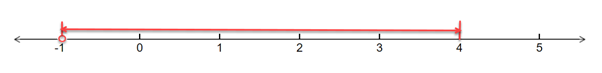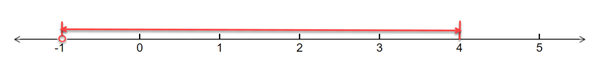# To solve the below inequality in terms of intervals and illustrate the solution set on the real number line - 1 &lt; 3 x + 4 ≤ 16### Single Variable Calculus: Concepts...

4th Edition
James Stewart
Publisher: Cengage Learning
ISBN: 9781337687805### Single Variable Calculus: Concepts...

4th Edition
James Stewart
Publisher: Cengage Learning
ISBN: 9781337687805

#### Solutions

Chapter A, Problem 16E
To determine

## To solve the below inequality in terms of intervals and illustrate the solution set on the real number line -   1<3x+4≤16

Expert Solution

The solution of the inequality is 1<x4 and the solution set on a real number line -### Explanation of Solution

Given: Inequality: 1<3x+416

Formula Used:

An inequality compares two values, showing if one is less than, greater than, or simply not equal to another value.

Real number line is the line whose points are the real numbers.

Calculation:

Given: Inequality equation is 1<3x+416

This inequality can be broken down into two inequalities:

1<3x+4 and 3x+416

Solving the firstinequality, we have:

Subtract (4) from both the sides -

14<3x+44

Solving further:

3<3x

Divideboth the sides by (3) , we have:

33<3x3

1<x

Solving the second inequality, we have:

3x+416

Subtract (4) from both the sides -

3x+44164

Solving further:

3x12

Divideboth the sides by (3) , we have:

3x3123

Solving further:

x4 ;

Combining both the inequalities, we have:

1<x4

Drawing the above inequality on a real number line, we have:Conclusion:

Hence, the solution of the inequality is 1<x4 and the solution set on a real number line -### Have a homework question?

Subscribe to bartleby learn! Ask subject matter experts 30 homework questions each month. Plus, you’ll have access to millions of step-by-step textbook answers!